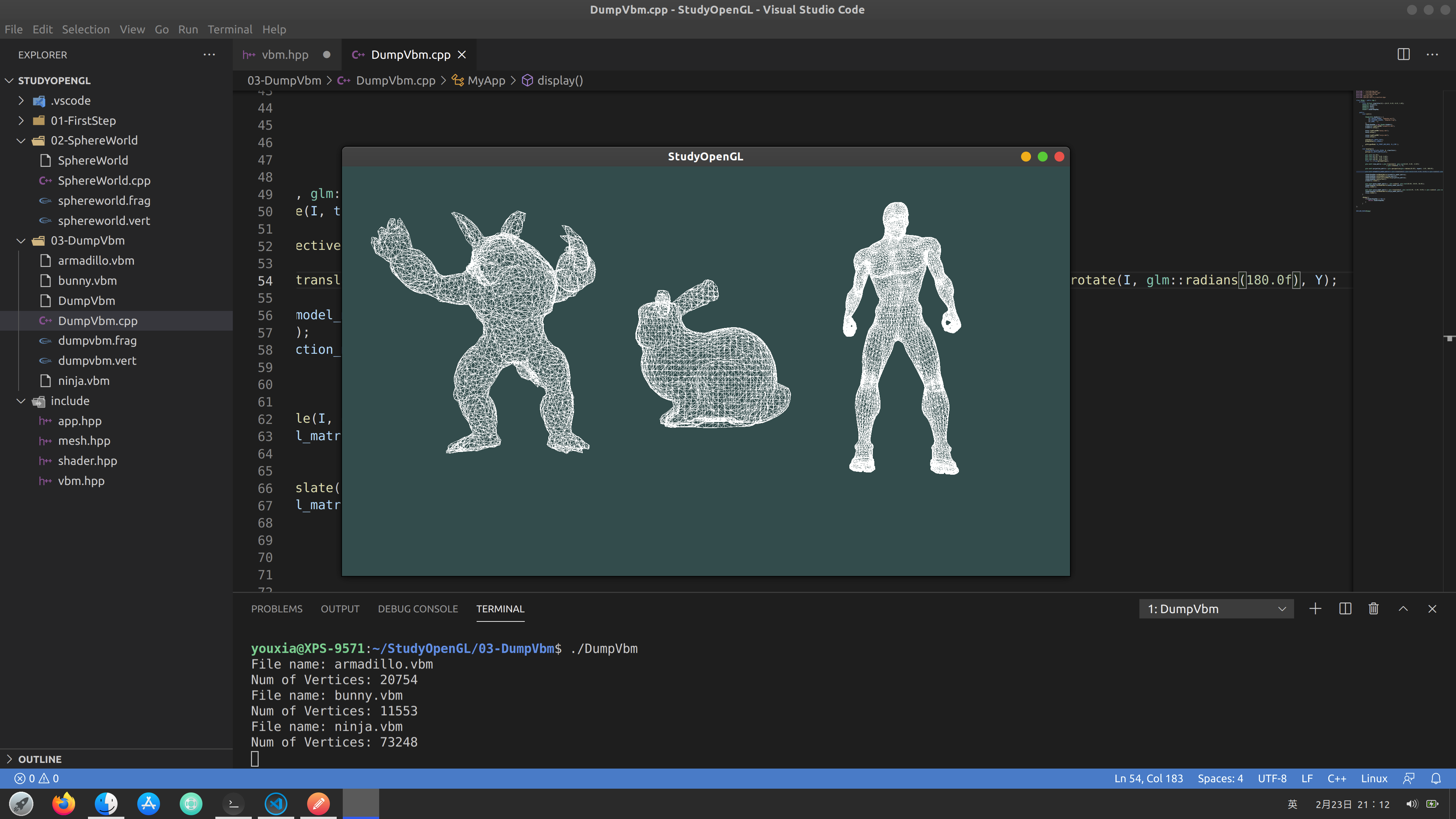## 编写我自己的 VbmObject 类

vbm.hpp 的完整代码如下：

``````#ifndef __VBM_H__
#define __VBM_H__

#include <glm/glm.hpp>
#include <glm/gtc/matrix_transform.hpp>
#include <vector>
#include <string>
#include <string.h>
#include <GL/glew.h>
#include <iostream>

{
unsigned int magic;
unsigned int size;
char name;
unsigned int num_attribs;
unsigned int num_frames;
unsigned int num_vertices;
unsigned int num_indices;
unsigned int index_type;
unsigned int num_materials;
unsigned int flags;

{
char name;
unsigned int type;
unsigned int components;
unsigned int flags;

{
unsigned int first;
unsigned int count;
unsigned int flags;

class VbmObject{
protected:
unsigned char* file_data;
unsigned char* vertex_data;
unsigned int vertex_num;
GLuint VAO, VBO;

public:
std::cout << "File name: " << filename << std::endl;
FILE * f = NULL;
f = fopen(filename, "rb");
if(f == NULL)
return false;

fseek(f, 0, SEEK_END);
size_t filesize = ftell(f);
fseek(f, 0, SEEK_SET);

file_data = new unsigned char [filesize];
fclose(f);

std::cout << "Num of Vertices: " << vertex_num << std::endl;

return true;
}

void setup(){
glCreateVertexArrays(1, &VAO);
glBindVertexArray(VAO);
glCreateBuffers(1, &VBO);
glBindBuffer(GL_ARRAY_BUFFER, VBO);
glNamedBufferStorage(VBO, 9*sizeof(GLfloat)*vertex_num, vertex_data, 0);
glVertexAttribPointer(0, 4, GL_FLOAT, GL_FALSE, 0, (void*)0);
glEnableVertexAttribArray(0);
glVertexAttribPointer(1, 3, GL_FLOAT, GL_FALSE, 0, (void*)(sizeof(GLfloat)*vertex_num*4));
glEnableVertexAttribArray(1);
glVertexAttribPointer(2, 2, GL_FLOAT, GL_FALSE, 0, (void*)(sizeof(GLfloat)*vertex_num*3));
glEnableVertexAttribArray(2);
}

void render(){
glBindVertexArray(VAO);
glDrawArrays(GL_TRIANGLES, 0, vertex_num);
}

~VbmObject(){
if(file_data != NULL){
delete file_data;
}
}
};

#endif
``````

DumpVbm.cpp 的完整内容如下：

``````#include "../include/app.hpp"
#include "../include/vbm.hpp"
#include <glm/glm.hpp>
#include <glm/gtc/matrix_transform.hpp>

class MyApp : public App {
private:
const GLfloat clearColor = {0.2f, 0.3f, 0.3f, 1.0f};
VbmObject bunny;
VbmObject ninja;

public:
void init(){

{GL_NONE, ""}
};

bunny.setup();

ninja.setup();

glEnable(GL_DEPTH_TEST);
glDepthFunc(GL_LEQUAL);

glPolygonMode( GL_FRONT_AND_BACK, GL_LINE );
}

void display(){
glClearBufferfv(GL_COLOR, 0, clearColor);
glClear(GL_DEPTH_BUFFER_BIT);

glm::mat4 I(1.0f);
glm::vec3 X(1.0f, 0.0f, 0.0f);
glm::vec3 Y(0.0f, 1.0f, 0.0f);
glm::vec3 Z(0.0f, 0.0f, 1.0f);
float t = (float)glfwGetTime();

glm::mat4 view_matrix = glm::translate(I, glm::vec3(0.0f, 0.0f, -5.0f))
* glm::rotate(I, t, Y);

glm::mat4 projection_matrix = glm::perspective(glm::radians(45.0f), aspect, 1.0f, 100.0f);

glm::mat4 armadillo_model_matrix = glm::translate(I, glm::vec3(-2.0f, 0.0f, 0.0f)) * glm::scale(I, glm::vec3(0.015f, 0.015f, 0.015f)) * glm::rotate(I, glm::radians(180.0f), Y);

glm::mat4 bunny_model_matrix =  glm::scale(I, glm::vec3(10.0f, 10.0f, 10.0f));
bunny.render();

glm::mat4 ninja_model_matrix = glm::translate(I, glm::vec3(2.0f, -1.0f, 0.0f)) * glm::scale(I, glm::vec3(0.015f, 0.015f, 0.015f));
ninja.render();
}

~MyApp(){
}
}

};

DECLARE_MAIN(MyApp)
``````

``````g++ DumpVbm.cpp -o DumpVbm -lGL -lglfw -lGLEW